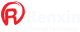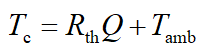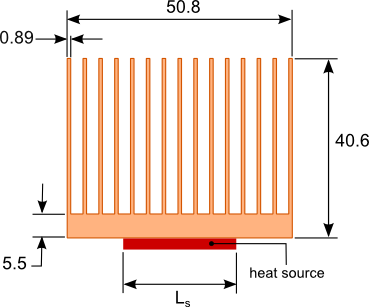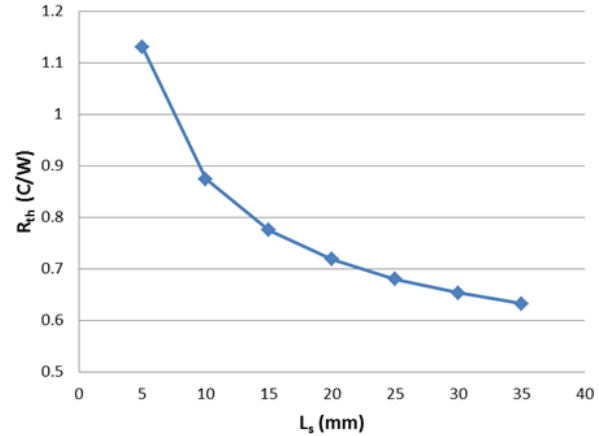#Misunderstandings about the selection of the Heatsink, Electronic engineers easily overlooked details
Misunderstandings about the selection of the Heatsink, Electronic engineers easily overlooked details
Misunderstandings about the selection of the Heatsink, Electronic engineers easily overlooked details
If you do not understand the basic factors affecting the performance of the heat sink, the heat sink may be insufficient to meet the selections cooling equipment cooling requirements. This will result in a loop iterative design and testing tasks, and ultimately delay the development process of the product. In assessing the general performance of the Heatsink, the following three common mistakes.

1:Thermal resistance of the heat sink provided by the manufacturer to evaluate the performance of the thermal

Heat sink thermal resistance is the most common method used in a particular application evaluating the performance of the heat sink. As shown in Equation (1) by the heat sink thermal resistance Rth multiplying the Q power dissipation of the cooling device, together with the ambient temperature Tamb, the temperature Tc of the housing apparatus can be determined. A typical method is to first select a heat sink using the formula 1 needed to calculate the thermal resistance of the heat sink, the thermal resistance of the heat sink and then select the calculated value or less,(Equation1)
Thermal resistance is typically provided by the manufacturer through the heat source of a square measured, usually having a size of 25.4 mm x 25.4 mm square heat source (heat consumption fixed) connected to the center of the bottom heat sink substrate, then measuring the temperature difference, the thermal resistance of the heat sink can be calculated by (Equation 1)

If the method of measuring the thermal resistance of the heat sink is select, there will often choose a  cooling solutions demand of non-compliance, since the thermal resistance of the heat sink is not a constant. The same heatsink heat resistance will vary with the size of the heatsink with respect to the reference plane. If you use a heat source is significantly less than the manufacturer used in the test the actual heat sink, the thermal resistance may be far higher than the value of the test manufacturer. This is due to the heat flows from the heat sink top surface of the source region, caused by the thermal diffusion resistance.Area of ​​the heatsink with respect to the substrate, the smaller the area of ​​the heat source, the heat  larger diffusion resistance, the greater the total thermal resistance of the heat sink. In the case of forced convection cooling, advection diffusion resistance to the most significant heat sink.( 1   schematic view of a heat sink geometry)
FIG 2 is a forced convection cooling, as the size of the heat sink thermal resistance curve of a heat source. Heatsink dimensions as shown in FIG. 76.2 mm in length of the heat sink, the source the same length and width, determined by Ls. Flow rate of 5 CFM, heat from the source is 59 W, the ambient temperature was 30 ℃.(2  -  Effect of heat resistance of Heatsink size)

The heatsink thermal resistance provided by the manufacturer is usually 76.2 mm in length for the Heatsink. Note that, when the cross section of the same heat sink, the heat sink thermal resistance does not vary with length (i.e., the depth of the Heatsink) increase linearly. With increasing length, since the ratio of the bottom area of ​​the heat sink area is reduced, the diffusion resistance will increase. This part of the heat transfer surface area increase brought gains were offset by increased diffusion resistance. Furthermore, as the length of the Heatsink, convection heat transfer efficiency of the Heatsink will be reduced.